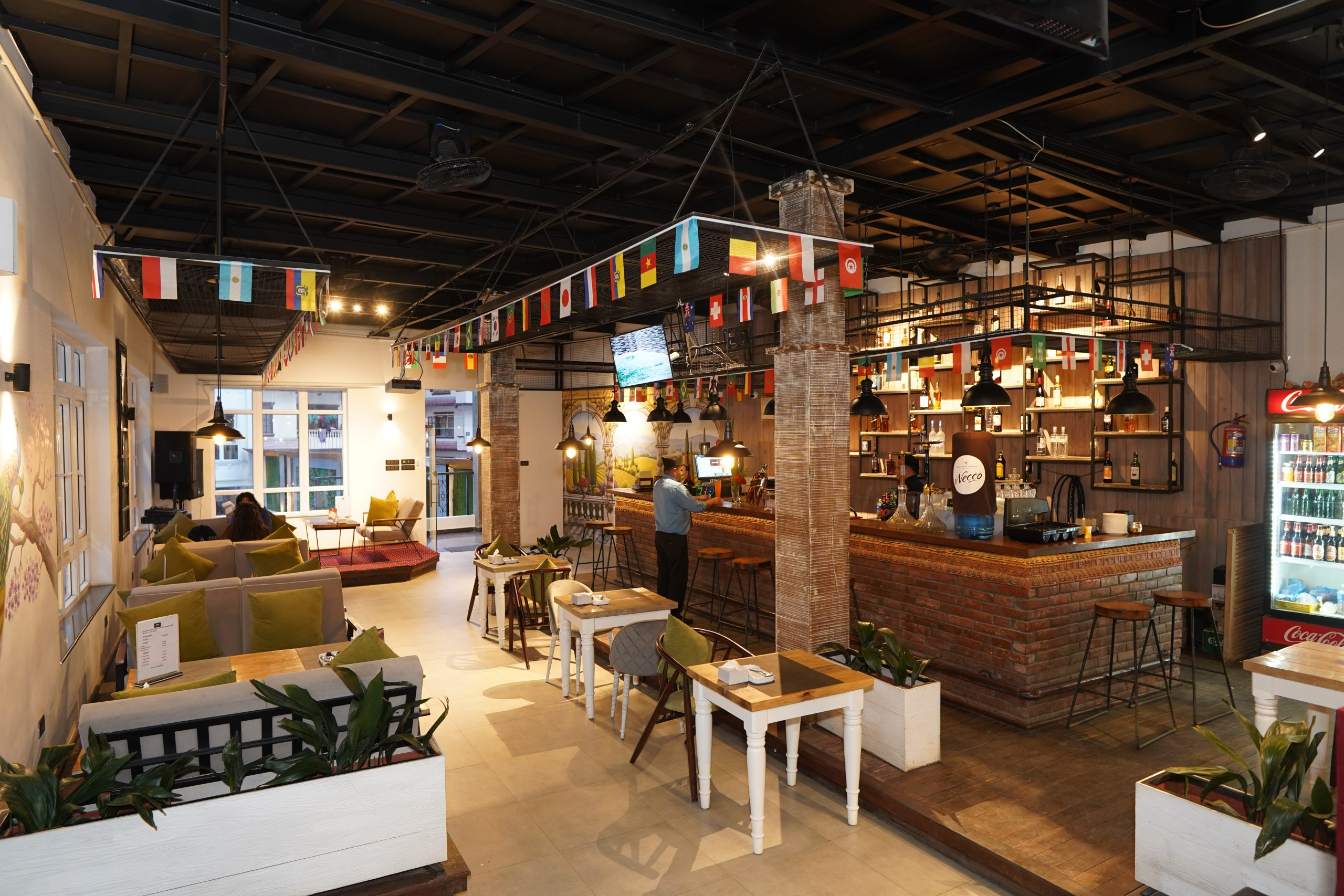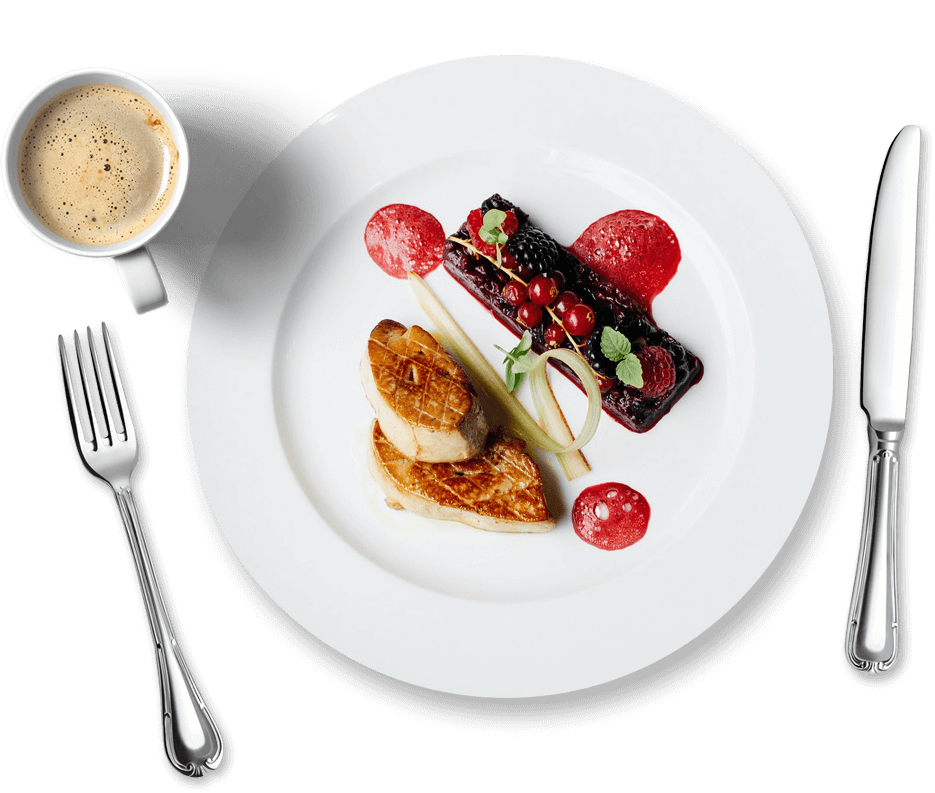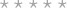Restaurant In Kathmandu | Restaurant Discount

## Welcome To### Mouth Watering Yumminess right at your plate!

The only satisfaction for a hungry tummy is a super-fast service with your yummy delicacies served just the way you like it!

Lotus Club gets it. And we are here to serve just that.

## Our Delightful

• All
• Alcoholic Beverages
• Breakfast
• Desert
• Drinks
• House Special
• Main Course
• Snacks
• Soup
• Starters

Rs. 15750
Rs. 4875
Rs. 3765
Rs. 2940
Rs. 2665
Rs. 4050
Rs. 3525
Rs. 3300
Rs. 4275
Rs. 4490
Rs. 2700
Rs. 2475
Rs. 1790

Rs. 6080
Rs. 4410
Rs. 5415
Rs. 3595
Rs. 2570
Rs. 2795
Rs. 2590
Rs. 3750
Rs. 2440
Rs. 2480
Rs. 2615
Rs. 4570
Rs. 2310
Rs. 2385
Rs. 2100
Rs. 2410
Rs. 2295
Rs. 3810
Rs. 2260
Rs. 2235
Rs. 2960
Rs. 425

Rs. 11770
Rs. 9640
Rs. 4220
Rs. 3860
Rs. 5595
Rs. 3115
Rs. 3470
Rs. 3140
Rs. 2490
Rs. 2205
Rs. 2890

Rs. 3480
Rs. 1075
Rs. 2990
Rs. 3480

Rs. 680
Rs. 670
Rs. 730
Rs. 1045
Rs. 550
Rs. 550

Rs. 500
Rs. 800
Rs. 30
Rs. 30
Rs. 550
Rs. 50
Rs. 100

Rs. 470
Rs. 590
Rs. 280
Rs. 580
Rs. 580
Rs. 560
Rs. 580
Rs. 395
Rs. 580
Rs. 225
Rs. 205

Rs. 530
Rs. 730
Rs. 580
Rs. 580

Rs. 615
Rs. 615
Rs. 590
Rs. 590
Rs. 590
Rs. 580
Rs. 580
Rs. 460
Rs. 470
Rs. 470
Rs. 450
Rs. 450
Rs. 590
Rs. 615

Rs. 295
Rs. 380
Rs. 495
Rs. 395

Rs. 390
Rs. 220
Rs. 350
Rs. 240
Rs. 375
Rs. 250
Rs. 260
Rs. 325
Rs. 390
Rs. 180
Rs. 125
Rs. 220
Rs. 220
Rs. 260
Rs. 220
Rs. 175
Rs. 475
Rs. 525
Rs. 575

Rs. 365
Rs. 595
Rs. 595
Rs. 365
Rs. 335
Rs. 365
Rs. 370
Rs. 390
Rs. 380
Rs. 375
Rs. 375
Rs. 350
Rs. 350
Rs. 375
Rs. 350
Rs. 380
Rs. 595
Rs. 370
Rs. 365
Rs. 415
Rs. 380

Rs. 200
Rs. 225
Rs. 215
Rs. 285
Rs. 250
Rs. 295
Rs. 200
Rs. 230
Rs. 220
Rs. 285
Rs. 250
Rs. 295

Rs. 375
Rs. 490

Rs. 525
Rs. 725
Rs. 680
Rs. 495
Rs. 540
Rs. 425
Rs. 535
Rs. 415
Rs. 550
Rs. 450
Rs. 510

Rs. 360
Rs. 495
Rs. 570

Rs. 285
Rs. 365
Rs. 365

Rs. 390
Rs. 390
Rs. 1100
Rs. 1850

Rs. 250
Rs. 290
Rs. 250
Rs. 290
Rs. 395

Rs. 210
Rs. 270
Rs. 260

Rs. 210
Rs. 270
Rs. 260

Rs. 220
Rs. 280
Rs. 270

Rs. 190
Rs. 235
Rs. 220

Rs. 190
Rs. 235
Rs. 220

Rs. 175
Rs. 210
Rs. 200

Rs. 220
Rs. 245
Rs. 240
Rs. 275

Rs. 260
Rs. 495
Rs. 560
Rs. 440
Rs. 495
Rs. 400

Rs. 210
Rs. 220
Rs. 230
Rs. 240
Rs. 230
Rs. 260
Rs. 350
Rs. 475

Rs. 350
Rs. 350
Rs. 300
Rs. 300
Rs. 290

Rs. 120
Rs. 150
Rs. 300
Rs. 125
Rs. 110
Rs. 140
Rs. 350
Rs. 250
Rs. 270
Rs. 325
Rs. 460
Rs. 325

Rs. 450
Rs. 350
Rs. 260

Rs. 600
Rs. 600
Rs. 450
Rs. 590
Rs. 525
Rs. 525
Rs. 575
Rs. 600

Rs. 250
Rs. 250
Rs. 325
Rs. 350
Rs. 350
Rs. 325
Rs. 425
Rs. 260
Rs. 125
Rs. 30

Rs. 495
Rs. 480
Rs. 480
Rs. 495
Rs. 600
Rs. 600
Rs. 780
Rs. 780
Rs. 770
Rs. 475
Rs. 285
Rs. 480
Rs. 350
Rs. 475
Rs. 325
Rs. 995
Rs. 1375

Rs. 2700
Rs. 1475
Rs. 2700
Rs. 1475
Rs. 1550
Rs. 850
Rs. 1950
Rs. 1150

Rs. 450
Rs. 450
Rs. 275

Rs. 290
Rs. 300
Rs. 300
Rs. 300
Rs. 295
Rs. 300
Rs. 420

Rs. 295
Rs. 295
Rs. 295
Rs. 280
Rs. 280

Rs. 150
Rs. 180
Rs. 180
Rs. 200
Rs. 200
Rs. 220
Rs. 220

Rs. 200
Rs. 160
Rs. 90
Rs. 80
Rs. 70
Rs. 50
Rs. 60
Rs. 140
Rs. 390
Rs. 130
Rs. 390
Rs. 130
Rs. 170
Rs. 190
Rs. 220
Rs. 230
Rs. 245
Rs. 95
Rs. 125
Rs. 250
Rs. 175
Rs. 365
Rs. 170
Rs. 190
Rs. 55

Rs. 190
Rs. 200
Rs. 220
Rs. 240
Rs. 200
Rs. 200
Rs. 200
Rs. 200
Rs. 200
Rs. 200

Rs. 120
Rs. 150
Rs. 100
Rs. 130
Rs. 150
Rs. 170
Rs. 160
Rs. 130
Rs. 160

## Discover## Location

Gongabu Bus Park, 350M ahead to Manmaiju Pul

## Working hour

Monday-Sunday: 10:00 – 22:00
View Cart Items (0)
QTY ITEMS PRICE